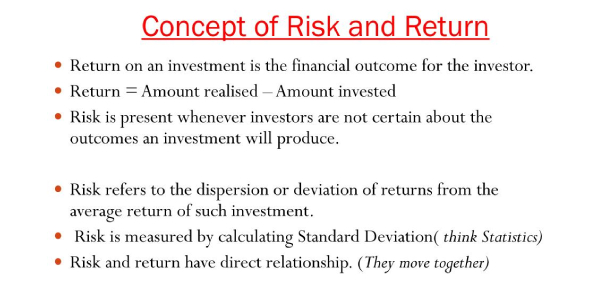# Concept Of Risk And Return Quiz

17 Questions | Attempts: 14878SettingsHere is an interesting concept of risk and return quiz that is designed to test your knowledge of this subject. There are a lot of things that people assess before they decide to invest in a project and this signifies an element of risk of making less money than intended. People take risks at different levels and it is believed that high-risk projects bring more return. Do take up the quiz and get to see just how much you know about risk in projects and its relation to the rate of return.

• 1.
The return may be thought of as _________________.
• A.

The growth in the value of an investment.

• B.

The risk associated with an investment.

• C.

Obtained only if the company pays dividends; without dividends, return is 0.

• D.

The process of returning the stock to the corporation that issued it.

• 2.
Dividends _____ the rate of return?
• A.

Decrease

• B.

Increase

• C.

Have no effect on

• D.

Increase the risk of

• 3.
A holding period _____________________.
• A.

Is always a year

• B.

Is always a month

• C.

Is always a quarter

• D.

Can be any length of time

• 4.
What does the standard deviation measure?
• A.

The gain on the investment.

• B.

The holding period

• C.

Risk

• D.

The amount of dividend.

• 5.
Think carefully about this one. Some investors will accept high-risk investments and some investors prefer low-risk investments.  What term best describes that situation?
• A.

Risk aversion

• B.

• C.

Risk tolerance

• D.

Rate of return

• 6.
An example of a specific risk:
• A.

A CEO is fired

• B.

A recession

• C.

A war

• D.

A presidential election

• 7.
In the financial markets, what is the DOW used for?
• A.

It is a measure of specific risk

• B.

It is a measure of market risk

• C.

It measures stock price levels for the entire market

• D.

It measures the level of dividends paid by various companies

• 8.
The DOW sample of stocks is considered a valid representation of the market because _____________________.
• A.

It is designed to include companies for major industries

• B.

It represents large amount (25%) of the value of all stocks traded

• C.

Both a and b

• D.

Neither a nor b

• 9.
How many stocks are included in the DOW?
• A.

20

• B.

30

• C.

100

• D.

500

• 10.
Which type of risk does diversification help to manage?
• A.

Specific

• B.

Market

• C.

Both a and b

• D.

Neither a nor b

• 11.
It is generally thought that an investor is fully diversified if she owns __ stocks.
• A.

1

• B.

5

• C.

20

• D.

30

• 12.
What risk does the standard deviation measure?
• A.

Specific

• B.

Market

• C.

Total

• D.

It doesn't - it measures return, not risk

• 13.
Investors profit from investments in stock by ______________.
• A.

Receiving dividends

• B.

Price growth

• C.

Both a and b

• D.

Neither a nor b

• 14.
A rational investor will invest in a risky stock if ________________.
• A.

They think they will earn a large return on the investment.

• B.

They have a high risk tolerance.

• C.

Both a and b

• D.

Neither a nor b

• 15.
Compared to the DOW, the S&P 500 is ________________________.
• A.

Is generally thought to represent a more valid sample

• B.

Includes small stocks

• C.

Includes less stocks than the DOW

• D.

Includes only foreign company stocks

• 16.
Which stock would an investor with a high risk tolerance prefer?
• A.

Stock A has a mean return of 7% and a standard deviation of 2%.

• B.

Stock B has a mean return of 7% and a standard deviation of 10%.

• C.

Stock C has a mean return of 12% and a standard deviation of 10%.

• D.

Stock D has a mean return of 12% and a standard deviation of 20%.

• 17.
The mean of 50 monthly returns return for a stock is 13%.  The standard deviation is 5%.  We can, therefore, be 68% certain that the return for any particular month is at least
• A.

13%

• B.

5%

• C.

18%

• D.

8%

## Related TopicsBack to top
×

Wait!
Here's an interesting quiz for you.